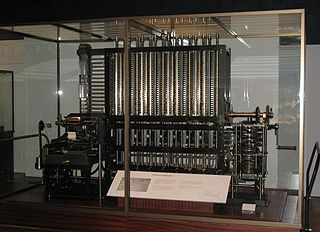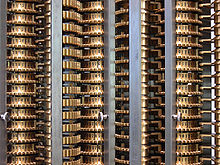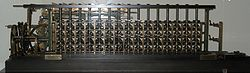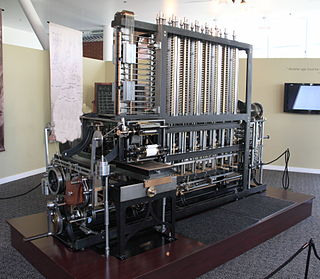# Difference engine

﻿
Difference engineThe London Science Museum's difference engine, built from Babbage's design. The design has the same precision on all columns, but when calculating converging polynomials, the precision on the higher-order columns could be lower. The Engine is not a replica (there never was one built during Babbage's lifetime); therefore this is the first one - the original.

A difference engine is an automatic, mechanical calculator designed to tabulate polynomial functions. Both logarithmic and trigonometric functions can be approximated by polynomials, so a difference engine can compute many useful sets of numbers.

## HistoryCloseup of the London Science Museum's difference engine showing some of the number wheels and the sector gears between columns. The sector gears on the left show the double-high teeth very clearly. The sector gears on the middle-right are facing the back side of the engine, but the single-high teeth are clearly visible. Notice how the wheels are mirrored, with counting up from left-to-right, or counting down from left-to-right. Also notice the metal tab between "6" and "7". That tab trips the carry lever in the back when "9" passes to "0" in the front during the add steps (Step 1 and Step 3).Per Georg Scheutz's third difference engine

J. H. Müller, an engineer in the Hessian army conceived the idea in a book published in 1786, but failed to find funding to progress this further.

In 1822, Charles Babbage proposed the use of such a machine in a paper to the Royal Astronomical Society on 14 June entitled "Note on the application of machinery to the computation of astronomical and mathematical tables". This machine used the decimal number system and was powered by cranking a handle. The British government was interested, since producing tables was time consuming and expensive and they hoped the difference engine would make the task more economical.

In 1823, the British government gave Babbage ₤1700 to start work on the project. The problem for Babbage was although the design was technically feasible, no one had built a mechanical device to these exacting standards before so the engine proved to be much more expensive than he had anticipated. By the time the government killed the project in 1842, they had given Babbage over ₤17,000. This was more than double the cost of a warship and the engine had still not been built. What Babbage did not, or was unwilling to, recognize was that the government was interested in economically produced tables, not the engine itself. The other issue that undermined the government’s confidence in the difference engine was Babbage had moved on to an analytical engine. By developing something better, Babbage had rendered the difference engine useless in the eyes of the government.

Babbage went on to design his much more general analytical engine but later produced an improved difference engine design (his "Difference Engine No. 2") between 1847 and 1849. Babbage was able to take advantage of ideas developed for the analytical engine to make the difference engine not only calculate more quickly but it could be made from fewer parts. Inspired by Babbage's difference engine plans, Per Georg Scheutz built several difference engines from 1855 onwards; one was sold to the British government in 1859. Martin Wiberg improved Scheutz's construction but used his device only for producing and publishing printed logarithmic tables.[citation needed]

Based on Babbage's original plans, the London Science Museum constructed a working difference engine No. 2 from 1989 to 1991, under Doron Swade, the then Curator of Computing. This was to celebrate the 200th anniversary of Babbage's birth. In 2000, the printer which Babbage originally designed for the difference engine was also completed. The conversion of the original design drawings into drawings suitable for engineering manufacturers' use revealed some minor errors in Babbage's design, which had to be corrected. Once completed, both the engine and its printer worked flawlessly, and still do. The difference engine and printer were constructed to tolerances achievable with 19th century technology, resolving a long-standing debate whether Babbage's design would actually have worked. (One of the reasons formerly advanced for the non-completion of Babbage's engines had been that engineering methods were insufficiently developed in the Victorian era.)

Although the "printer" is here referred to as such, its primary purpose is to produce stereotype plates for use in printing presses; Babbage's intention being that the Engine's results be conveyed directly to mass printing. Babbage recognized that errors in previous tables were not the result of human calculating errors but from human error in the printing process. The printer's paper output is mainly a means of checking the Engine's performance.

In addition to funding the construction of the output mechanism for the Science Museum's Difference Engine No. 2, Nathan Myhrvold commissioned the construction of a second complete Difference Engine No. 2, which has been on exhibit at the Computer History Museum in Mountain View, California starting on 10 May 2008 and continuing through the end of 2011.

## Operation

The difference engine consists of a number of columns, numbered from 1 to N. The machine is able to store one decimal number in each column. The machine can only add the value of a column n + 1 to column n to produce the new value of n. Column N can only store a constant, column 1 displays (and possibly prints) the value of the calculation on the current iteration.

The engine is programmed by setting initial values to the columns. Column 1 is set to the value of the polynomial at the start of computation. Column 2 is set to a value derived from the first and higher derivatives of the polynomial at the same value of X. Each of the columns from 3 to N is set to a value derived from the (n − 1) first and higher derivatives of the polynomial.

### Timing

In the Babbage design, one iteration i.e. one full set of addition and carry operations happens once for four rotations of the crank. Odd and even columns alternately perform an addition in one cycle. The sequence of operations for column n is thus:

1. Count up, receiving the value from column n + 1 (Addition step)
2. Perform carry propagation on the counted up value
3. Count down to zero, adding to column n − 1
4. Reset the counted down value to its original value

Steps 1,2,3,4 occur for every odd column while steps 3,4,1,2 occur for every even column.

### Steps

Each iteration creates a new result, and is accomplished in four steps corresponding to four complete turns of the handle shown at the far right in the picture below. The four steps are:

• Step 1. All even numbered columns (2,4,6,8) are added to all odd numbered columns (1,3,5,7) simultaneously. An interior sweep arm turns each even column to cause whatever number is on each wheel to count down to zero. As a wheel turns to zero, it transfers its value to a sector gear located between the odd/even columns. These values are transferred to the odd column causing them to count up. Any odd column value that passes from "9" to "0" activates a carry lever.
• Step 2. Carry propagation is accomplished by a set of spiral arms in the back that poll the carry levers in a helical manner so that a carry at any level can increment the wheel above by one. That can create a carry, which is why the arms move in a spiral. At the same time, the sector gears are returned to their original position, which causes them to increment the even column wheels back to their original values. The sector gears are double-high on one side so they can be lifted to disengage from the odd column wheels while they still remain in contact with the even column wheels.
• Step 3. This is like Step 1, except it is odd columns (3,5,7) added to even columns (2,4,6), and column one has its values transferred by a sector gear to the print mechanism on the left end of the engine. Any even column value that passes from "9" to "0" activates a carry lever. The column 1 value, the result for the polynomial, is sent to the attached printer mechanism.
• Step 4. This is like Step 2, but for doing carries on even columns, and returning odd columns to their original values.

### Subtraction

The engine represents negative numbers as ten's complements. Subtraction amounts to addition of a negative number. This works in exactly the same manner that modern computers perform subtraction, known as two's complement.

## Method of differencesFully operational difference engine at the Computer History Museum in Mountain View, California

The principle of a difference engine is Newton's method of divided differences. If the initial value of a polynomial (and of its finite differences) is calculated by some means for some value of X, the difference engine can calculate any number of nearby values, using the method generally known as the method of finite differences. It may be illustrated with a small example. Consider the quadratic polynomial

p(x) = 2x2 − 3x + 2

and suppose we want to tabulate the values p(0), p(1), p(2), p(3), p(4) etc. The table below is constructed as follows: the second column contains the values of the polynomial, the third column contains the differences of the two left neighbors in the second column, and the fourth column contains the differences of the two neighbors in the third column:

x p(x) = 2x2 − 3x + 2 diff1(x) = ( p(x+1) - p(x) ) diff2(x) = ( diff1(x+1) - diff1(x) )
0 2 -1 4
1 1 3 4
2 4 7 4
3 11 11
4 22

Notice how the numbers in the third values-column are constant. This is no mere coincidence. In fact, if you start with any polynomial of degree n, the column number n + 1 will always be constant. This crucial fact makes the method work, as we will see next.

We constructed this table from the left to the right, but now we can continue it from the right to the left down a diagonal in order to compute more values of our polynomial.

To calculate p(5) we use the values from the lowest diagonal. We start with the fourth column constant value of 4 and copy it down the column. Then we continue the third column by adding 4 to 11 to get 15. Next we continue the second column by taking its previous value, 22 and adding the 15 from the third column. Thus p(5) is 22+15 = 37. In order to compute p(6), we iterate the same algorithm on the p(5) values: take 4 from the fourth column, add that to the third column's value 15 to get 19, then add that to the second column's value 37 to get 56, which is p(6).

This process may be continued ad infinitum. The values of the polynomial are produced without ever having to multiply. A difference engine only needs to be able to add. From one loop to the next, it needs to store 2 numbers in our case (the last elements in the first and second columns); if we wanted to tabulate polynomials of degree n, we'd need enough storage to hold n numbers.

Babbage's difference engine No. 2, finally built in 1991, could hold 8 numbers of 31 decimal digits each and could thus tabulate 7th degree polynomials to that precision. The best machines from Scheutz were able to store 4 numbers with 15 digits each.[citation needed]

## Initial values

The initial values of columns can be calculated by first manually calculating N consecutive values of the function and by backtracking, i.e. calculating the required differences.

Col 10 gets the value of the function at the start of computation f(0). Col 20 is the difference between f(1) and f(0)...

If the function to be calculated is a polynomial function, expressed as$f(x) = a_n x^n + a_{n-1} x^{n-1} + \cdots + a_2 x^2 + a_1 x + a_0 \,$

the initial values can be calculated directly from the constant coefficients a0, a1,a2, ..., an without calculating any data points. The initial values are thus:

• Col 10 = a0
• Col 20 = a1 + a2 + a3 + a4 + ... + an
• Col 30 = 2a2 + 6a3 + 14a4 + 30a5 + ...
• Col 40 = 6a3 + 36a4 + 150a5 + ...
• Col 50 = 24a4 + 240a5 + ...
• Col 60 = 120a5 + ...
• ...

### Use of derivatives

Many commonly used functions are analytic functions, which can be expressed as power series, for example as a Taylor series. The initial values can be calculated to any degree of accuracy; if done correctly the engine will give exact results for first N steps. After that, the engine will only give an approximation of the function.

The Taylor series expresses the function as a sum of its derivatives. For many functions the higher derivatives are trivial to obtain; the sine function at 0 has derivates of 0 or + / − 1 for all derivates. Setting 0 as the start of computation we get the simplified Maclaurin series$\sum_{n=0}^{\infin} \frac{f^{(n)}(0)}{n!} (x)^{n}$

The same method of calculating the initial values from the coefficients can be used as for polynomial functions. The polynomial constant coefficients will now have the value$a_n \equiv \frac{f^{(n)}(0)}{n!}$

### Curve fitting

The problem with the methods described above is that errors will accumulate and the series will tend to diverge from the true function. A solution which guarantees a constant maximum error is to use curve fitting. A minimum of N values are calculated evenly spaced along the range of the desired calculations. Using a curve fitting technique like Gaussian reduction a N-1th degree polynomial interpolation of the function is found. With the optimized polynomial, the initial values can be calculated as above.

Wikimedia Foundation. 2010.

### Look at other dictionaries:

• Difference Engine —   [engl.], Differenzmaschine …   Universal-Lexikon

• Difference Engine — Eine Differenzmaschine (englisch difference engine) ist ein mechanischer Computer zum Lösen polynomialer Funktionen. Da jede stetig differenzierbare Funktion durch ein Polynom angenähert werden kann (Approximation), ist eine derartige Maschine… …   Deutsch Wikipedia

• Difference engine — Eine Differenzmaschine (englisch difference engine) ist ein mechanischer Computer zum Lösen polynomialer Funktionen. Da jede stetig differenzierbare Funktion durch ein Polynom angenähert werden kann (Approximation), ist eine derartige Maschine… …   Deutsch Wikipedia

• Difference Engine — ▪ calculating machine       an early calculating machine, verging on being the first computer, designed and partially built during the 1820s and 30s by Charles Babbage (Babbage, Charles). Babbage was an English mathematician and inventor; he… …   Universalium

• difference engine — Babidžo analitinė mašina statusas T sritis informatika apibrėžtis Pirmoji universali mechaninė skaičiavimo mašina. Analitinę skaičiavimo mašiną ir kompiuterių konstravimo principus sukūrė Kembridžo universiteto profesorius Čarlzas Babidžas… …   Enciklopedinis kompiuterijos žodynas

• difference engine — noun A mechanical special purpose computer used to generate tables of values of polynomials …   Wiktionary

• Difference Engine — von Charles Babbage 1812 entwickelte und 1922 gebaute Rechenmaschine für astronomische Tabellen …   Acronyms

• Difference Engine — von Charles Babbage 1812 entwickelte und 1922 gebaute Rechenmaschine für astronomische Tabellen …   Acronyms von A bis Z

• The Difference Engine — For the machine designed by Charles Babbage, see Difference engine. The Difference Engine   …   Wikipedia

• The Difference Engine — Die Differenzmaschine (engl. The Difference Engine) ist eine Alternativweltgeschichte von William Gibson und Bruce Sterling. Es ist ein herausragendes Beispiel des Steampunk Subgenre. Im Viktorianischen England des Romans regiert die industrielle …   Deutsch Wikipedia